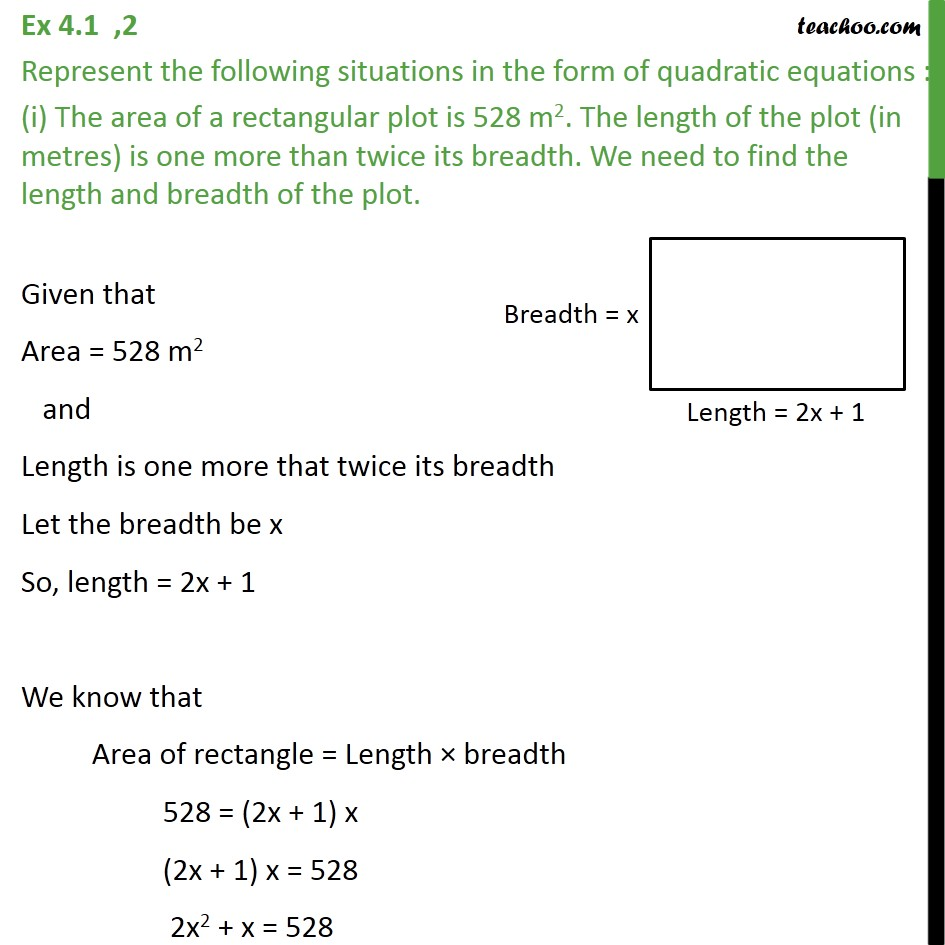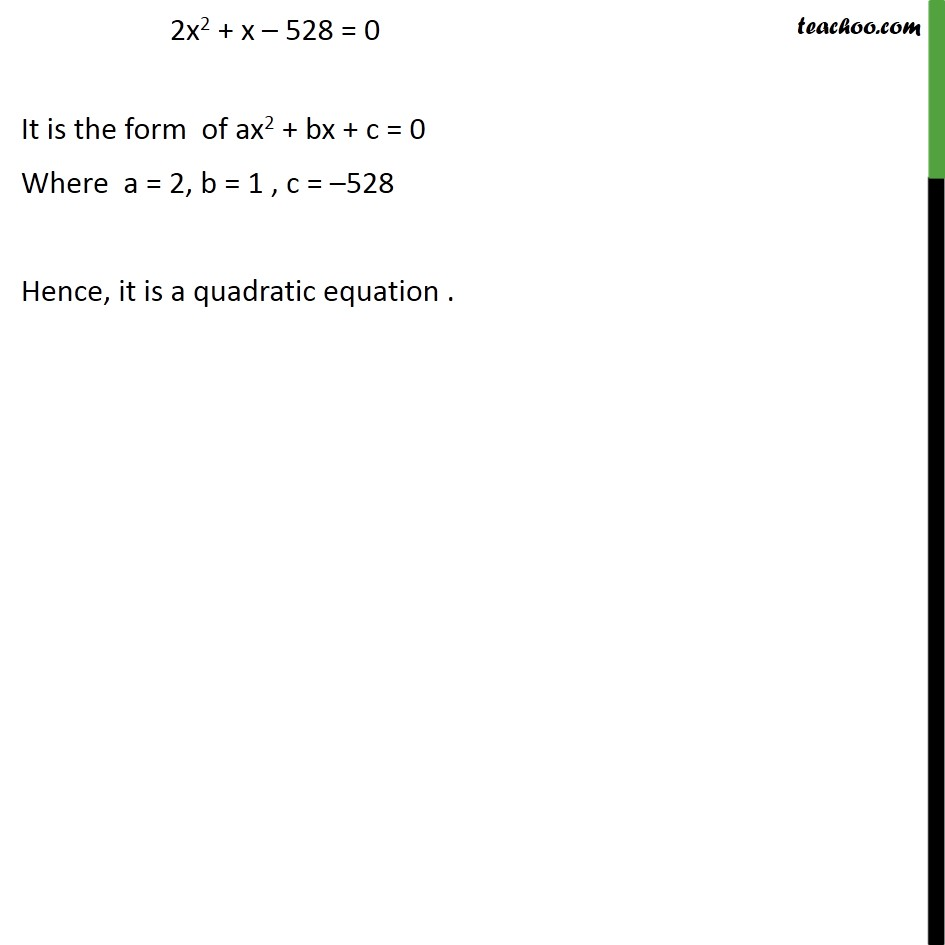1. Chapter 4 Class 10 Quadratic Equations (Term 2)
2. Concept wise

Transcript

Ex 4.1 ,2 Represent the following situations in the form of quadratic equations : (i) The area of a rectangular plot is 528 m2. The length of the plot (in metres) is one more than twice its breadth. We need to find the length and breadth of the plot. Given that Area = 528 m2 and Length is one more that twice its breadth Let the breadth be x So, length = 2x + 1 We know that Area of rectangle = Length breadth 528 = (2x + 1) x (2x + 1) x = 528 2x2 + x = 528 2x2 + x 528 = 0 It is the form of ax2 + bx + c = 0 Where a = 2, b = 1 , c = 528 Hence, it is a quadratic equation .